# Given A and b to the​ right, write the augmented matrix for the linear system that corresponds to the matrix equation Axequals=b. Then solve the system and write the solution as a vector. Aequals=left...

Given A and b to the​ right, write the augmented matrix for the linear system that corresponds to the matrix equation Axequals=b. Then solve the system and write the solution as a vector. Aequals=left bracket Start 3 By 3 Matrix 1st Row 1st Column 1 2nd Column 3 3rd Column negative 2 2nd Row 1st Column negative 2 2nd Column negative 2 3rd Column 0 3rd Row 1st Column 5 2nd Column 2 3rd Column 6 EndMatrix right bracket 1 3 −2 −2 −2 0 5 2 6 ​, bequals=left bracket Start 3 By 1 Matrix 1st Row 1st Column 4 2nd Row 1st Column negative 4 3rd Row 1st Column 19 EndMatrix right bracket 4 −4 19 Write the augmented matrix for the linear system that corresponds to the matrix equation Upper A Bold x equals Bold bAx=b. Select the correct choice below and fill in any answer boxes within your choice. A. left bracket Start 3 By 4 Matrix 1st Row 1st Column nothing 2nd Column nothing 3rd Column nothing 4st Column nothing 2nd Row 1st Column nothing 2nd Column nothing 3rd Column nothing 4st Column nothing 3rd Row 1st Column nothing 2nd Column nothing 3rd Column nothing 4st Column nothing EndMatrix right bracket 11 33 −2negative 2 44 −2negative 2 −2negative 2 00 −4negative 4 55 22 66 1919 Your answer is correct.B. left bracket Start 3 By 3 Matrix 1st Row 1st Column nothing 2nd Column nothing 3rd Column nothing 2nd Row 1st Column nothing 2nd Column nothing 3rd Column nothing 3rd Row 1st Column nothing 2nd Column nothing 3rd Column nothing EndMatrix right bracket nothing nothing nothing nothing nothing nothing nothing nothing nothing Solve the system and write the solution as a vector. Select the correct choice below and fill in any answer boxes within your choice. A. xequals=left bracket Start 2 By 1 Matrix 1st Row 1st Column nothing 2nd Row 1st Column nothing EndMatrix right bracket left bracket Start 3 By 1 Matrix 1st Row 1st Column Superscript 2nd Row 1st Column 3rd Row 1st Column Subscript EndMatrix right bracket B. xequals=left bracket Start 3 By 1 Matrix 1st Row 1st Column nothing 2nd Row 1st Column nothing 3rd Row 1st Column nothing EndMatrix right bracket nothing left bracket Start 3 By 1 Matrix 1st Row 1st Column Superscript 2nd Row 1st Column 3rd Row 1st Column Subscript EndMatrix right bracket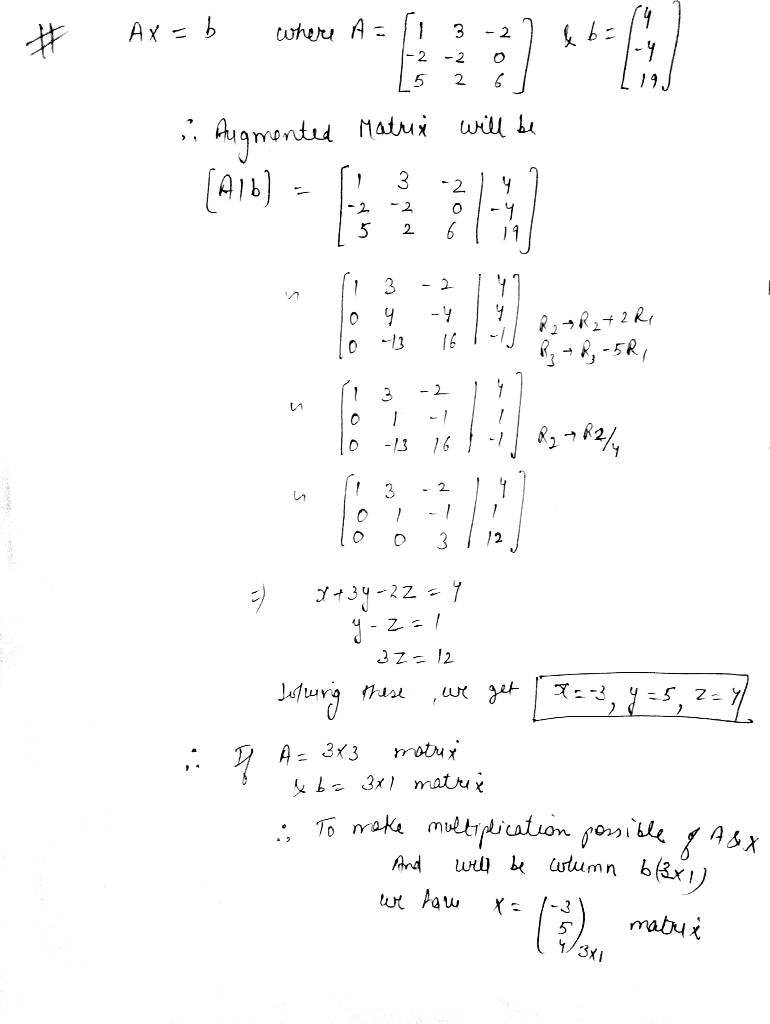##### Add Answer of: Given A and b to the​ right, write the augmented matrix for the linear system that corresponds to the matrix equation Axequals=b. Then solve the system and write the solution as a vector. Aequals=left...
Similar Homework Help Questions
• ### Determine ModifyingBelow lim With x right arrow c Superscript plus​f(x), ModifyingBelow lim With x right arrow c Superscript minus​f(x), and ModifyingBelow lim With x right arrow c​f(x), if it exists....

Determine ModifyingBelow lim With x right arrow c Superscript plus​f(x), ModifyingBelow lim With x right arrow c Superscript minus​f(x), and ModifyingBelow lim With x right arrow c​f(x), if it exists. cequals3​,​ f(x)equals left brace Start 2 By 2 Matrix 1st Row 1st Column 4 minus x 2nd Column x less than 3 2nd Row 1st Column StartFraction x Over 3 EndFraction plus 1 2nd Column x greater than 3 EndMatrix ModifyingBelow lim With x right arrow c Superscript plus​f(x)equals nothing...

• ### Given the following system of linear equations 1. 2xi + 4x2 + 8 x3 + x. +2x,3 a) Write the augmented matrix that represents the system b) Find a reduced row echelon form (RREF) matrix that is row...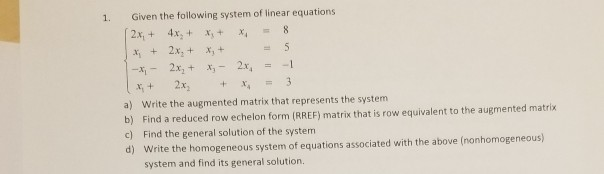Given the following system of linear equations 1. 2xi + 4x2 + 8 x3 + x. +2x,3 a) Write the augmented matrix that represents the system b) Find a reduced row echelon form (RREF) matrix that is row equivalent to the augmented matrix c) Find the general solution of the system d) Write the homogeneous system of equations associated with the above (nonhomogeneous) system and find its general solution. Given the following system of linear equations 1. 2xi + 4x2...

• ### Use an augmented matrix to solve the linear system

Use an augmented matrix to solve the linear system. x+4y-2z=3 Step #1 [1 4 -2: 3] x+3y+7z=1 [1 3 7 :1] 2x+9y+z=8 [2 9 1 :8]

• ### intersection in planes for the last three rows Write a system of linear equations and the row reduced echelon form (RREF) of the corresponding augmented matrix that meets the requirements describe...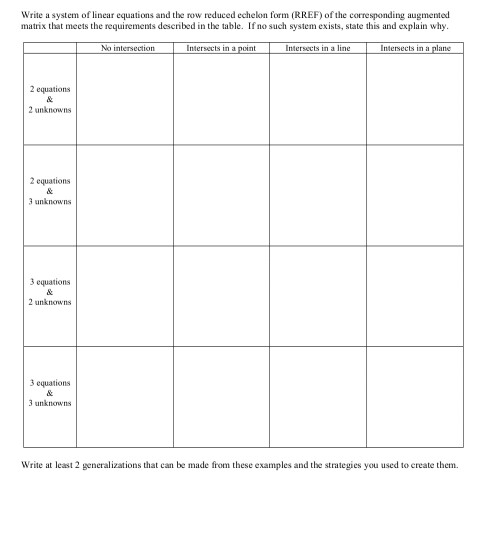intersection in planes for the last three rows Write a system of linear equations and the row reduced echelon form (RREF) of the corresponding augmented matrix that meets the requirements described in the table. Ifno such system exists, state this and explain why. Intersects in a point No intersection Intersects in a line Intersects in a plane 2 equations 2 unknowns 2 equations 3 unknowns 3 equations 2 unknowns 3 equations unknowns Write at least 2 generalizations that can be...

• ### Write the system first as a vector equation and then as a matrix equation. 4x1- x2 = 6 2x110x2 2 6x1- X2=1 Write the sy...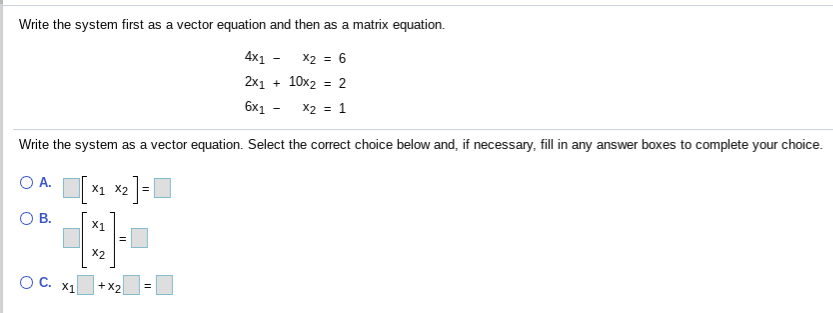Write the system first as a vector equation and then as a matrix equation. 4x1- x2 = 6 2x110x2 2 6x1- X2=1 Write the system as a vector equation. Select the correct choice below and, if necessary, fill in any answer boxes to complete your choice. X2 Write the system first as a vector equation and then as a matrix equation. 4x1- x2 = 6 2x110x2 - 2 6X1- X2=1 Write the system as a matrix equation. Select the correct...

• ### 3x0+1x2 + ! 040-2 8] [3 11. The augmented matrix for the linear system of equations in the unknowns a, y, z has red...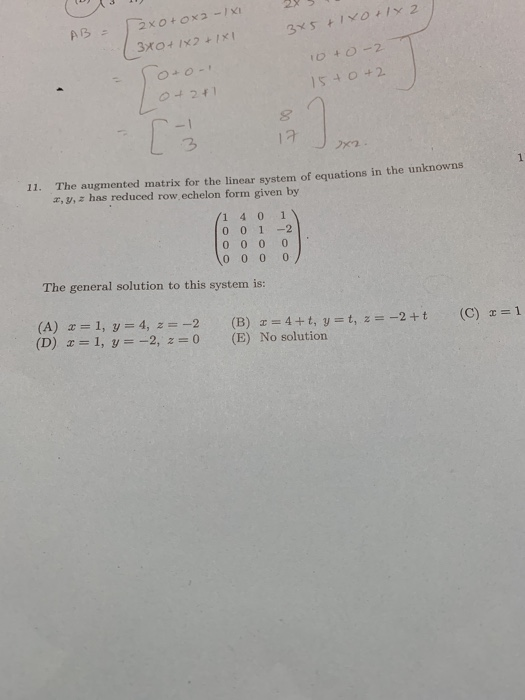3x0+1x2 + ! 040-2 8] [3 11. The augmented matrix for the linear system of equations in the unknowns a, y, z has reduced row,echelon form given by 1401 0 01 -2 The general solution to this syste is (D) x = 1, y =-2, z = 0 (E) No solution 3x0+1x2 + ! 040-2 8] [3 11. The augmented matrix for the linear system of equations in the unknowns a, y, z has reduced row,echelon form given by 1401...

• ### Write the augmented matrix of the systemWrite the augmented matrix of the system

• ### From the following augmented matrix, first write the system of equations that represents the augmented matrix and then create a real-world word problem that would represent these equations and their unknowns

From the following augmented matrix, first write the system of equations that represents the augmented matrix and then create a real-world word problem that would represent these equations and their unknowns. Be creative. Do not use word problems that are in the assignments or course material. é1 1 1ùéxù é100ù ê5 -3 0úêyú = ê 10ú ë0 3 4ûëzû ë290û

• ### For a given system the system A-matrix is given by 4 3 2 5 367 1 A = 2 7 5 3 5 3 2 The matrix of left eigen vectors U a...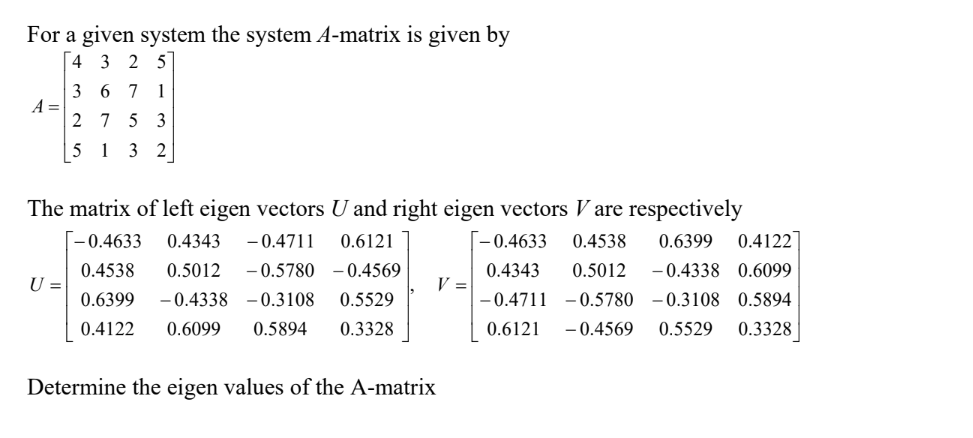For a given system the system A-matrix is given by 4 3 2 5 367 1 A = 2 7 5 3 5 3 2 The matrix of left eigen vectors U and right eigen vectors Vare respectively -0.4633 -0.4633 0.4122 0.4343 -0.4711 0.6121 0.4538 0.6399 -0.5780 0.4538 0.5012 0.4569 0.4343 0.5012 -0.4338 0.6099 U = V = -0.4711 0.5780 0.6399 -0.4338 -0.3108 0.5529 -0.3108 0.5894 -0.4569 0.3328 0.4122 0.6099 0.5894 0.3328 0.6121 0.5529 Determine the eigen values of the...

• ### can someone help me solve these question? thanks!!! 4. Find the general solution of the system whose augmented matrix is given by 1 -3 0 0-2 (a) 0 1 0 0 -1 0 0 0 94 1 3 -2 0 20 0 2 6 -5 -2 4-3-1 0 0 5...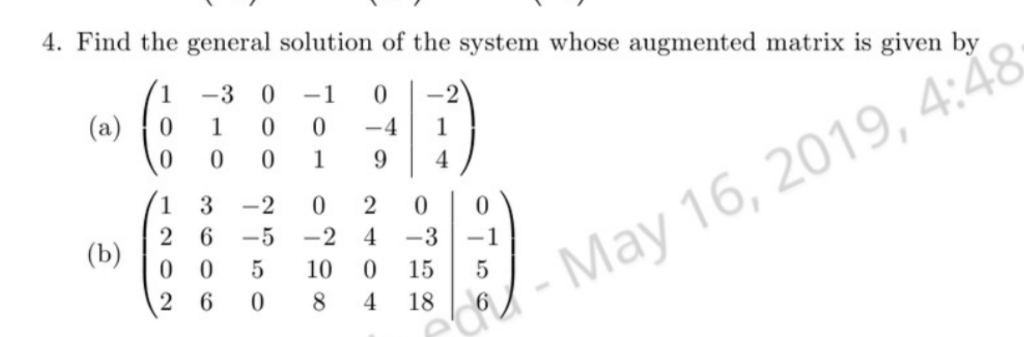can someone help me solve these question? thanks!!! 4. Find the general solution of the system whose augmented matrix is given by 1 -3 0 0-2 (a) 0 1 0 0 -1 0 0 0 94 1 3 -2 0 20 0 2 6 -5 -2 4-3-1 0 0 5 10 0 15 5 May 16, 2019, 4:48 2 6 08418 6 4. Find the general solution of the system whose augmented matrix is given by 1 -3 0 0-2...

Need Online Homework Help?